# Learn the concept of Arrays in Linux Shell Scripting

0
14984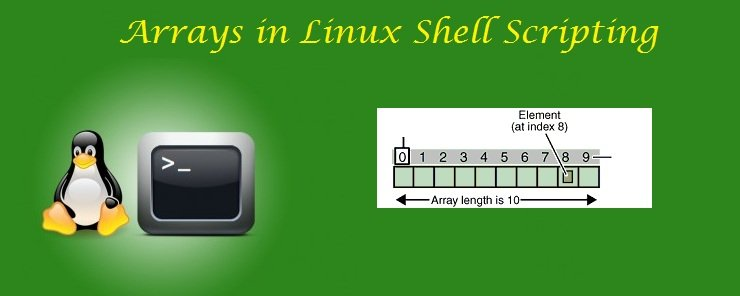An Array is a data structure that stores a list (collection) of objects (elements) that are accessible using zero-based index. In Linux shells, arrays are not bound to a specific data type; there is no array of data type integer, and array of data type float. An array can contain an integer value in one element, and a string value in the element next to it.

Initializing Arrays
An array can be defined and initialized by initializing its individual elements, one per statement:

```array1=12
array1=Ahmed
array1=76
```

An alternate way is to initialize the entire array in one step:

```player=(Alessandro DelPiero 10 Juventus)
countries=(Brazil Italy Germany Argentina England France Spain)
```

A third way is to use the Shell commands expansions. For example, the wild card * represents every file in a given directory.

`CurDirFiles=(*)`

This is equivalent to saying:

`CurDirFiles=( calc2.sh calc.sh factorial.sh first.sh functions1.sh kill_process.sh rename_files.sh strlen.sh uppercase.sh )`
`lowercase=({a..z})`

This is equivalent to saying:

`lowercase=(a b c d e f g h i j k l m n o p q r s t u v w x y z)`
`users=(user0{1..9})`

This is equivalent to saying:

`users=(user01 user02 user03 user04 user05 user06 user07 user08 user09)`
`rev_UPPERCASE=({Z..A})`

This is equivalent to saying:

`rev_UPPERCASE=(Z Y X W V U T S R Q P O N M L K J I H G F E D C B A)`

Accessing Array Elements
To Access an array element, use the following syntax:

`\${array_name[index]}`

Where: array_name is the name of the array to access its element.
index is a zero-based address used to reference a specific array element.

For example:
To print the first element in the users array: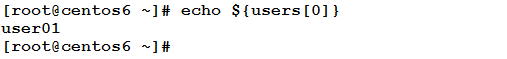To assign the value of the 4th element to the variable var: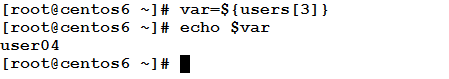Filling an Array by User’s Input
An array can be filled using user’s provided input values in two ways:

• By using the read command to repeatedly prompt the user to enter the next element.
• By providing the values of the elements as command-line arguments.

The following two examples will show both methods.

Example
This example prompts the user to enter elements for an array, one after another, and stores them in an array named inputarray.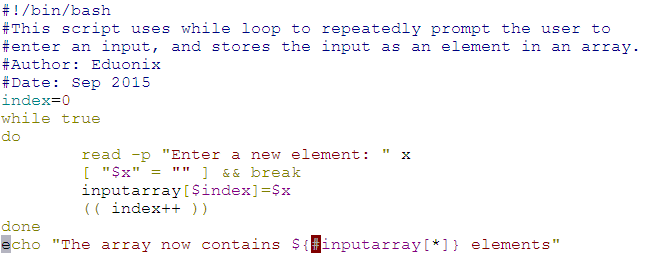To see it in action, execute the script as follows: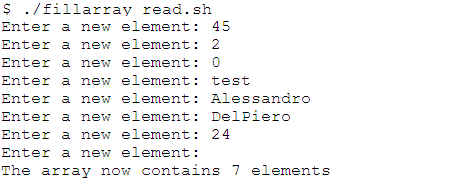Now, let’s explain things: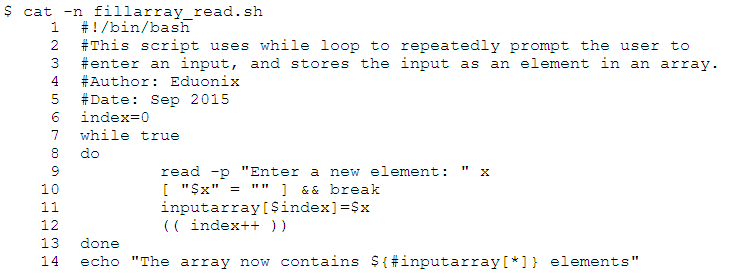• Line 6: initializes the array index to 0.
• Line 7: starts an endless loop.
• Lines 8 and 13: mark the start and end of the loop body.
• Lines 9-12: The loop body:
• Line 9: prompts the user to enter a value for a new array element, and stores the input value in variable x.
• Line 10: checks whether x (the input) is an empty string or not. If so, it executes the break statement to exit the loop.
• Line 11: If not, this line is executed. It stores the value of x in the inputarray.
• Line 12: increments the array index.
• Line 14: prints the length (number of elements) in the resulting array.

Very easy, isn’t it?!Example
In this example, we are going to fill an array using values provided as command-line arguments at execution.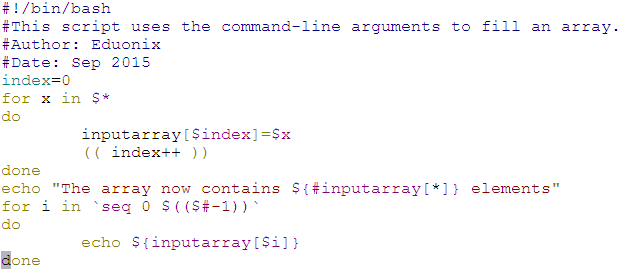Let’s execute it and see what will happen: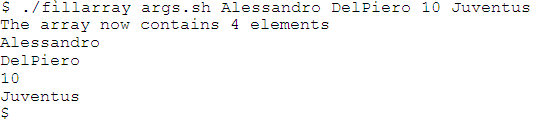Now, to the explanation.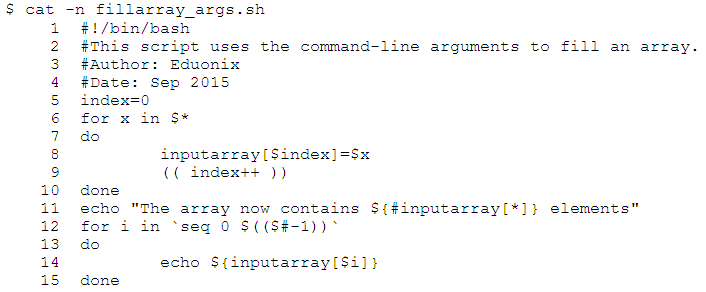• Line 6: uses a for loop that will iterate over the list of arguments passed to the script at execution (stored in the \$* special variable).
• Lines 7 and 10: mark the start and end of the loop body.
• Lines 8-9: The loop body:
• Line 8: assigns the current value of the loop variable x to the array element at the current value of the variable index.
• Line 9: increments the array index.
• Line 11: prints the resulting array length.
• Lines 12-15: prints the array elements, one per line.

Arrays Operations
Arrays have some useful operations like the getting the length of an array, and printing the entire array.

Obtaining Array Length
To get the length (number of elements) of an array, use the following syntax:

`\${#array_name[*]}`

For example: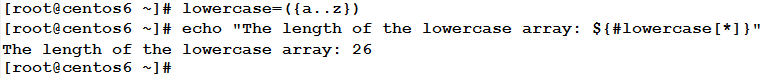Needless to say that the alphabetical letters are 26, so the array containing all the lowercase letters will be 26 elements long.

Printing an Entire Array
To print all the elements in an array: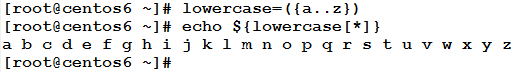Copying Arrays
To copy the contents of an array into another one, I prefer to use the following syntax:

`newarray=(\${orig_array[*]})`

Where orig_array is the original array to be copied.
newarray is the array to which the original array is copied.

Example
To copy the contents of the lowercase array into another array called array2: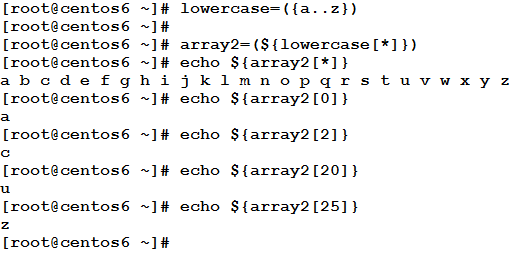Concatenating Arrays
To concatenate two or more arrays into one, use the following syntax:

`newarray=(\${array1[*]} \${array2[*]})`

where: newarray is the result of concatenation.
Take care of the space between array1 and array2.

Example
We have two arrays: lowercase and uppercase. From their names, the first contains lowercase letters, while the other contains uppercase letters. We need to concatenate them, creating a new array called alphabet. To achieve this consider the following statements: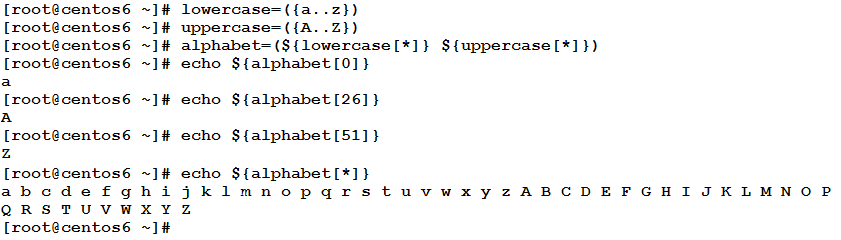* * * * * *

In this article, we have talked about arrays. Arrays are collections of objects that are accessible via index. Arrays in Linux shell are not restricted to a specific type, so one array could contain integer elements, and string elements as well. I hope you find this article useful.

See you in the next article.